Joint Hypothesis Tests with Complex Alternatives, the Chi-Bar-Square Statistic

Silvapulle and Sen (2004) propose a test statistic for testing hypotheses where the null or the alternative hypothesis or both involve inequalities. You can test special cases of these hypotheses with the JOINT option in the ESTIMATE and the LSMESTIMATE statement. Consider theestimable functionsand the hypotheses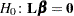and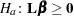. The alternative hypothesis defines a convex cone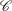at the origin. Suppose that under the null hypothesis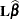follows a multivariate normal distribution with mean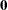and variance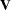. The restricted alternative prevents you from using the usualor chi-square test machinery, since the distribution of the test statistic under the alternative might not follow the usual rules. Silvapulle and Sen (2004) coined a statistic that takes into account the projection of the observed estimate onto the convex cone formed by the alternative parameter space. This test statistic is called the chi-bar-square statistic, and-values are obtained by simulation; see, in particular, Chapter 3.4 in Silvapulle and Sen (2004).

Briefly, let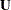be a multivariate normal random variable with meanand variance matrix. The chi-bar-square statistic is the random variable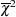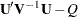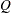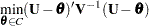and it can be motivated by a geometric argument. The quadratic form in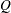is the-projection ofonto the cone. Suppose that this projected point is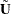. If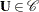, then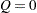and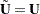. Ifis completely outside of the cone, thenis a point on the surface of the cone. Similarly,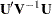is the length of the segment from the origin toin the-space with norm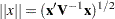. If you apply the Pythagorean theorem, you can see that the chi-bar-square statistic measures the length of the segment from the origin to the projected pointin.

To calculate-values for chi-bar-square statistics, a simulation-based approach is taken. Consider again the set ofestimable functionswith estimate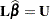and variance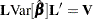.

First, the observed value of the statistic is computed as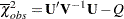Then,independent random samples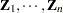are drawn from an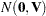distribution and the following chi-bar-statistics are computed for the sample: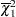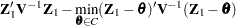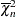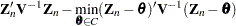The-value is estimated by the fraction of simulated statistics that are greater than or equal to the observed value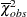.

Notice that unlessis interior to the cone, finding the value ofrequires the solution to a quadratic optimization problem. Whenis large, or when many simulations are requested, the computation of-values for chi-bar-square statistics might require considerable computing time.